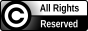Ragnar Nevries

## Efficient domination and polarity

Universität Rostock, 2014

https://doi.org/10.18453/rosdok_id00001431

Abstract: The thesis considers the following graph problems: Efficient (Edge) Domination seeks for an independent vertex (edge) subset D such that all other vertices (edges) have exactly one neighbor in D. Polarity asks for a vertex subset that induces a complete multipartite graph and that contains a vertex of every induced P_3. Monopolarity is the special case of Polarity where the wanted vertex subset has to be independent. These problems are NP-complete in general, but efficiently solvable on various graph classes. The thesis sharpens known NP-completeness results and presents new solvable cases.

doctoral thesis   freeaccess